# Ordering Decimals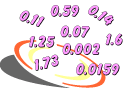Example 1: The Glosser Family drove to a gasoline station in their neighborhood. The station has three gas pumps, each marked in price per gallon. Which pump has the lowest price per gallon? Which pump has the highest price per gallon?

\$1.79,  \$1.96,  \$1.61

Analysis: We know that 1.79 < 1.96 and that 1.79 > 1.61. Writing one decimal beneath the other in order, we get:

 1 . 6 1least 1 . 7 91 . 9 6greatest

Answer: The pump marked \$1.61 has the lowest price per gallon. The pump marked \$1.96 has the highest price per gallon.

In the example above, we ordered three decimal numbers from least to greatest by comparing them two at a time. Let's look at some more examples.

Example 2: Order these decimals from least to greatest:  0.5629,  0.5621,  0.6521

Let's examine these decimals in our place-value-chart.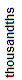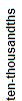0 . 5 6 2 9 0 . 5 6 2 1 0 . 6 5 2 1

Now let's order these decimals from least to greatest without our place-value chart. We will do this by comparing two decimals at a time.

 0 . 5 6 2 1 0 . 5 6 2 9 0 . 6 5 2 1

Answer: Ordering these decimals from least to greatest we get:  0.5621,  0.5629,  0.6521.

In the examples above, the decimals in each problem had the same number of digits. Thus, they lined up nicely, one beneath the other. Let's look at some examples in which the decimals presented have a different number of decimal digits.

Example 3: Order these decimals from least to greatest:  6.01,  0.601,  6.1

Let's start by writing one decimal beneath the other in their original order. Note that these three decimals have a different number of decimal digits.

 6 . 0 1 0 0 . 6 0 1 6 . 1 0 0

Next, examine each decimal, writing one or more zeros to the right of the last digit, so that all decimals have the same number of decimal digits.

 6 . 0 1 0 0 . 6 0 1 6 . 1 0 0

Now we can compare two decimals at a time.

 6 . 0 1 0 0 . 6 0 1 6 . 1 0 0

From least to greatest, we get:   0.6010,  6.010,  6.100.

Answer: Ordering these decimals from least to greatest we get:  0.601,  6.01,  6.1.

Sometimes it is helpful to place a number in a circle to the right of each decimal you are trying to order. This is done in Example 4.

Example 4: Order these decimals from least to greatest:  3.87,  3.0875,  3.87502,  3.807

We have been asked to order four decimal numbers. Let's start by writing one decimal beneath the other in their original order.

 3 . 8 7 0 0 0 3 . 0 8 7 5 0 3 . 8 7 5 0 2 3 . 8 0 7 0 0

Next, examine each decimal, writing one or more zeros to the right of the last digit, so that all decimals have the same number of decimal digits.

 3 . 8 7 0 0 0 3 . 0 8 7 5 0 3 . 8 7 5 0 2 3 . 8 0 7 0 0

Now we can compare two decimals at a time. We will write a number in a circle next to each decimal to denote its order.

 3 . 8 7 0 0 0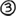3 . 0 8 7 5 0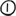3 . 8 7 5 0 23 . 8 0 7 0 0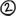From least to greatest, we get:  3.08750,  3.80700,  3.87000,  3.87502

Answer: Ordering these decimals from least to greatest we get:  3.0875,  3.807,  3.87,  3.87502

Example 5: Order these decimals from least to greatest:  5.364,  6.0364,  5.36,  5.00364,  5.40364

We have been asked to order five decimal numbers. Let's start by writing one decimal beneath the other in their original order. Next, examine each decimal, writing one or more zeros to the right of the last digit, as needed.

 5 . 3 6 4 0 0 6 . 0 3 6 4 0 5 . 3 6 0 0 0 5 . 0 0 3 6 4 5 . 4 0 3 6 4

Now we can compare two decimals at a time. We will write a number in a circle next to each decimal to denote its order.

 5 . 3 6 4 0 06 . 0 3 6 4 05 . 3 6 0 0 05 . 0 0 3 6 45 . 4 0 3 6 4From least to greatest, we get:  5.00364,  5.36000,  5.36400,  5.40364,  6.03640

Answer: Ordering these decimals from least to greatest we get:  5.00364,  5.36,  5.364,  5.40364,  6.0364

Let's look at some non-routine problems that involve comparing and ordering decimals.

Example 6: Write 3 decimals between 4.35 and 4.36 in order from least to greatest.

Analysis: We need to write a zero in the thousandths place for each of the given numbers.

 4 . 3 5 0 4 . 3 6 0
Now we must find 3 numbers that are between the given numbers. We can use any digit between 1 and 9.
 4 . 3 5 0 4 . 3 5 1 4 . 3 5 2 4 . 3 5 3 4 . 3 5 4 4 . 3 5 5 4 . 3 5 6 4 . 3 5 7 4 . 3 5 8 4 . 3 5 9 4 . 3 6 0
The question asks us to write 3 decimals between 4.35 and 4.36 in order from least to greatest. We can choose any 3 numbers in red from above as long as they are in order from least to greatest. Accordingly, our answers will vary. Below are some sample answers.

Sample Answer 1: 4.351,  4.352,  4.353

Sample Answer 2: 4.354,  4.356,  4.358

Example 7: Write 3 decimals between 7.418 and 7.419 in order from least to greatest.

Analysis: We need to write a zero in the ten-thousandths place for each of the given numbers.

 7 . 4 1 8 0 7 . 4 1 9 0
Now we must find 3 numbers that are between the given numbers. We can use any digit between 1 and 9.
 7 . 4 1 8 0 7 . 4 1 8 1 7 . 4 1 8 2 7 . 4 1 8 3 7 . 4 1 8 4 7 . 4 1 8 5 7 . 4 1 8 6 7 . 4 1 8 7 7 . 4 1 8 8 7 . 4 1 8 9 7 . 4 1 9 0
The question asks us to write 3 decimals between 7.418 and 7.419 in order from least to greatest. We can choose any 3 numbers in red from above as long as they are in order from least to greatest. Accordingly, our answers will vary. Below are some sample answers.

Sample Answer 1: 7.4182,  7.4183,  7.4184

Sample Answer 2: 7.4185,  7.4187,  7.4189

Example 8: Write the smallest possible decimal between zero and one that uses the digits 5, 0, 4, 1, 9, and 6 exactly once.

Example 9: Write the greatest possible decimal between zero and one that uses the digits 9, 0, 2, 7, 3 and
5 exactly once.

Answer: .975320 (without a leading zero) or 0.97532 (with a leading zero). Note that these two decimals are equivalent.

Summary: When ordering decimals, first write one decimal beneath the other in their original order. Then compare them two at a time. When ordering four or more decimals, it is helpful to write a number in a circle next to each to order them.

### Exercises

 1. Which of the following is the smallest decimal number?0.49810.520.48910.6  RESULTS BOX:
 2. Which of the following is the largest decimal number?0.02310.2310.030.2 RESULTS BOX:
 3. Which of the following choices lists these decimals in order from least to greatest:  0.910,  0.091,  0.9?0.9,  0.091, 0.9100.910, 0.9, 0.0910.091, 0.9, 0.910None of the Above RESULTS BOX:
 4. Which of the following choices lists these decimals in order from least to greatest:  3.45,  3.0459,  3.5,  3.4059?3.0459, 3.4059, 3.45, 3.53.4059, 3.5, 3.0459, 3.453.5, 3.45, 3.4059, 3.0459None of the above. RESULTS BOX:
 5. Which of the following choices lists these decimals in order from least to greatest:  7.102,  7.0102,  7.012,  7.00102,  7.102021?7.102021, 7.00102, 7.012, 7.0102, 7.1027.00102, 7.0102, 7.012, 7.102, 7.1020217.102021, 7.102, 7.012, 7.0102, 7.00102None of the above. RESULTS BOX: